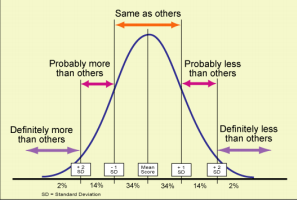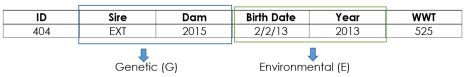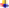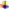×
Get Full Access to CSU - ANEQ 328 - Class Notes - Week 15
Get Full Access to CSU - ANEQ 328 - Class Notes - Week 15

×

CSU / Science / ANEQ 328 / What is a population in statistics?

# What is a population in statistics? Description

##### Description: Material covered in lecture on 4/12/16-4/14/16.
8 Pages 45 Views 1 Unlocks
Reviews

ANEQ 328 Foundations In Animal Genetics

## What is a population in statistics?Week 13 Class Notes

(4/12/16-4/14/16)

Must Know Statistics: Especially To Understand Quantitative Genetics

∙ Important Background Information

o Population

▪ A breeding population of animals.

o Value

▪ A numeric value applied to an individual as opposed to a

population.

⮚ Phenotypic Trait

o Trait

▪ A genetically determined characteristics.

⮚ Trait doesn’t always equal phenotype.

o Simple Trait

▪ When a gene is controlled by 1 or a few loci.

## What is a value in statistics?▪ Can be qualitative or categorical.

⮚ Ex. The coat color of a horse is only controlled by a few loci.

o Complex Trait

▪ When a gene is controlled by many loci.

▪ Can be quantitative or polygenic.

⮚ Ex. Milk production in cows in controlled by many loci.

o Mean

▪ The average of a set of numbers.

⮚ To calculate: Just add up all the numbers, then divide by how  many numbers there are.

∙ Normal Distribution (Bell Curve)

o The statistical distribution that appears graphically as a symmetric, bell shaped curve.

F## What is a simple trait in statistics?Don't forget about the age old question of What are the three categories of crime?

r

e

q

u

e

n

c

y

Category

ANEQ 328 Foundations In Animal Genetics

Week 13 Class Notes

(4/12/16-4/14/16)

▪ About 66% of the animal population will fall within the Standard

Deviation of the mean.

▪ About 95% of the animal population will fall with 2 Standard

Deviations of the mean.

▪ 99% of the animal population will fall within 3 Standard Deviations of  the mean.

∙ Mean (��)

o The average of a set of numbers.

▪ To calculate: Just add up all the numbers, then divide by how many  numbers there are.

⮚ Units: ��

o Using the following data to calculate the mean of the weaning and  yearling weights.

Calf ID

Weaning Weight (lbs; X)

Yearling Weight (lbs; Y)

CSU 2001

500

1100

CSU 2002

475

1050

CSU 2003

525

1150

We also discuss several other topics like What is the difference between the embodied emotion in the body against the brain?
If you want to learn more check out What are the three essential elements of a hypothesis?

▪ Weaning Weight Mean Calculation

(500 + 475 + 425)

3=1400

3= 466.67��

▪ Yearling Weight Mean Calculation

(1100 + 1050 + 1150)

3=3300

3= 1100��

∙ Variance (��2)

o Differences among individuals within a population.

▪ Equation: ��2 =∑(��−����)2

��−1or ��2 =(��1−����)2+(��2−����)2+(��3−����)2……

��−1

o Using the following data to calculate the variance of the weaning and  yearling weights.

Calf ID

Weaning Weight (lbs; X)

Yearling Weight (lbs; Y)

CSU 2001

500

1100

CSU 2002

475

1050

CSU 2003

525

1150

We also discuss several other topics like What is the content of schachter-singer's “two factor” theory?

o We know that the number in our population is 3 calves, so n=3.

ANEQ 328 Foundations In Animal Genetics

Week 13 Class Notes

(4/12/16-4/14/16)

▪ Weaning Weight Variance Calculation

(500 − 500)2 + (475 − 500)2 + (525 − 500)2

3 − 1=(��)2 + (−25)2 + (25)2

2=0 + 625 + 625

2=1250

2= 625������2 = ����2

▪ Yearling Weight Variance Calculation

(1100 − 1100)2 + (1050 − 1100)2 + (1150 − 1100)2

3 − 1=(��)2 + (−50)2 + (50)2

2=0 + 2500 + 2500 If you want to learn more check out What is flavivirus?

2=5000

2= 2500������2 =����2

∙ Standard Deviation (��)

o The average deviation from the mean.

▪ The square root of the variance.

⮚ Equation: √��2

o Using the following data to calculate the standard variation of the weaning  and yearling weights.

Calf ID

Weaning Weight (lbs; X)

Yearling Weight (lbs; Y)

CSU 2001

500

1100

CSU 2002

475

1050

CSU 2003

525

1150

o Based on previous calculations we know that ����2 = 625������2 and ����2 = 2500������2. Don't forget about the age old question of What does benevolent sexism mean?

▪ Weaning Weight Standard Deviation Calculation

����2 = 625������2 = √625 = 25������=����

▪ Yearling Weight Standard Deviation Calculation

����2 = 2500������2 = √2500 = 50������ = ����

∙ Standard Error (SE)

o Equation: ��√��or ���������������� ������������������

√������������ ���� ��������������������

o Using the following data to calculate the standard error of the weaning  and yearling weights.

ANEQ 328 Foundations In Animal Genetics

Week 13 Class Notes

(4/12/16-4/14/16)

Calf ID

Weaning Weight (lbs; X)

Yearling Weight (lbs; Y)

CSU 2001

500

1100

CSU 2002

475

1050

CSU 2003

525

1150

o Based on previous calculations we know that ���� = 25������ and ���� = 50������ and  the number in our population is 3 calves, so n=3.

▪ Weaning Weight Standard Error Calculation

���� = 25������ =25

√3= 15������ = ����. 500 ± 15������

▪ Yearling Weight Standard Error Calculation

���� = 50������ =50

√3= 30������ = ����. 1100 ± 30������

∙ Covariance (Cov(x,y))

o How two traits or values vary together in a population.

▪ Equation: (��1−����)(��1−����)+(��2−����)(��2−����)+(��3−����)(��3−����).

��−1

⮚ Has no units, just a positive or negative number.

o Using the following data to calculate the covariance of the weaning and  yearling weights.

Calf ID

Weaning Weight (lbs; X)

Yearling Weight (lbs; Y)

CSU 2001

500

1100

CSU 2002

475

1050

CSU 2003

525

1150

o We know that the number in our population is 3 calves, so n=3.

▪ Weaning Weight and Yearling Weight Covariance Calculation

(500 − 500)(1100 − 1100) + (475 − 500)(1050 −1100) + (525 − 500)(1150 − 1100) 3 − 1=2500

2= 1250������

o Covariation

▪ 1.) Positive or Negative Number

▪ 2.) Correlation shows the strength of a relationship.

▪ 3.) Regression show how much (amount).

ANEQ 328 Foundations In Animal Genetics

Week 13 Class Notes

(4/12/16-4/14/16)

∙ Correlation (r)

o A measure of strength of the relationship between two variables. ▪ Equation: ������ =(Cov(x,y))

��������

▪ If the correlation is between 0 and 1 it demonstrates a strong

relationship.

▪ If the correlation is between 0 and -1 it demonstrates a weak

relationship.

o Using the following data to calculate the correlation of the weaning and  yearling weights.

Calf ID

Weaning Weight (lbs; X)

Yearling Weight (lbs; Y)

CSU 2001

500

1100

CSU 2002

475

1050

CSU 2003

525

1150

o Based on previous calculations we know that (Cov(x, y)) = 1250lbs and ���� = 25������ and ���� = 50������.

▪ Weaning Weight and Yearling Weight Standard Correlation Calculation

(Cov(x, y)) = 1250lbs

(���� = 25������)(���� = 50������)=1250

(25)(50)= 1

∙ Regression (b)

o The expected or average change in one variable (y) per unit change in  another (x).

▪ Equation: ����∗�� =(Cov(x,y))

����2

o Using the following data to calculate the regression of the weaning and  yearling weights.

Calf ID

Weaning Weight (lbs; X)

Yearling Weight (lbs; Y)

CSU 2001

500

1100

CSU 2002

475

1050

CSU 2003

525

1150

o Based on previous calculations we know that (Cov(x, y)) = 1250lbs and ����2 = 625������.

▪ Weaning Weight and Yearling Weight Standard Regression Calculation

(Cov(x, y)) = 1250lbs

����2 = 625������ =1250

625 = 2

❖ For every 1lb increase in weaning weight, yearling

weight increases an average of 2 lbs.

ANEQ 328 Foundations In Animal Genetics

Week 13 Class Notes

(4/12/16-4/14/16)

Introduction to the Genetic Model for Quantitative Traits

∙ Important Background Information

o Population

▪ A breeding population of animals.

o Value

▪ A numeric value applied to an individual as opposed to a

population.

⮚ Phenotypic Trait

o Trait

▪ A genetically determined characteristics.

⮚ Trait doesn’t always equal phenotype.

o Simple Trait

▪ When a gene is controlled by 1 or a few loci.

▪ Can be qualitative or categorical.

⮚ Ex. The coat color of a horse is only controlled by a few loci.

o Complex Trait

▪ When a gene is controlled by many loci.

▪ Can be quantitative or polygenic.

⮚ Ex. Milk production in cows in controlled by many loci.

∙ Genetic Model for Quantitative Traits

o Equation: P=��+G+E

▪ P= Phenotypic Value

⮚ The performance of an individual animal for a specific trait.

▪ ��= Population mean

⮚ The average phenotypic value for the specific trait for all

animals in the population.

▪ G= Genotypic value

⮚ The genotypic values of the individual for the specific trait.

▪ E= Environmental Effect

⮚ The environmental effects on the individual’s performance for  the trait.

∙ Genetic Model for Quantitative Traits Including Breeding Value and Gene Combination Value o Equation: P=��+BV+GCV+E

▪ P= Phenotypic Value

⮚ The performance of an individual animal for a specific trait.

▪ ��= Population mean

⮚ The average phenotypic value for the specific trait for all

animals in the population.

▪ BV= Breeding Value

⮚ The value of the individual as a parent, (the sum of the

independent genotypes).

ANEQ 328 Foundations In Animal Genetics

Week 13 Class Notes

(4/12/16-4/14/16)

❖ Ex. A= +5, a= -5

✔ AA= Value of 10

✔ aa= Value of -10

✔ Aa= Value of 0

▪ GCV= Gene Combination Value

⮚ An individual’s genotypic value of gene interaction.

▪ E= Environmental Effect

⮚ The environmental effects on the individual’s performance for

the trait.

∙ Progeny Difference (PD)

o The expected difference between the mean performance of the  individual’s progeny and the mean performance of all progeny.

▪ Equation: PD= ½BV

▪ BV= Breeding Value

⮚ The value of the individual as a parent, (the sum of the

independent genotypes).

∙ Genetic Prediction and Its Datao The bigger the data the more accurate the EPD’s are.

▪ EPD’s get better as time goes on.

⮚ By adding multiple traits, animal models, and pedigree’s to an

EPD it makes it more accurate the younger the animal is.

∙ Genetic Model For Repeated Quantitative Traits

o Producing Ability (PA)

▪ The performance potential of an individual for a repeated trait.

⮚ Equation: PA= ��+BV+GCV+Ep+Et

❖ ��= Population mean

✔ The average phenotypic value for the specific

trait for all animals in the population.

❖ BV= Breeding Value

✔ The value of the individual as a parent, (the sum

of the independent genotypes).Ex. A= +5, a= -5

o AA= Value of 10

ANEQ 328 Foundations In Animal Genetics

Week 13 Class Notes

(4/12/16-4/14/16)

o aa= Value of -10

o Aa= Value of 0

❖ GCV= Gene Combination Value

✔ An individual’s genotypic value of gene

interaction.

❖ Ep= Permanent Environmental Effect

✔ An environmental effect that permanently influences an individual’s performance for a repeated trait.Ex. Increase or decrease in performance. ❖ Et= Temporary Environmental Effect

✔ An environmental effect that influences a single  performance record of an individual but does  not permanently affect an individual’s

performance potential for a repeated trait.Ex. Dry summer results in low numbers

being collected for the particular trait.

Page ExpiredIt looks like your free minutes have expired! Lucky for you we have all the content you need, just sign up here